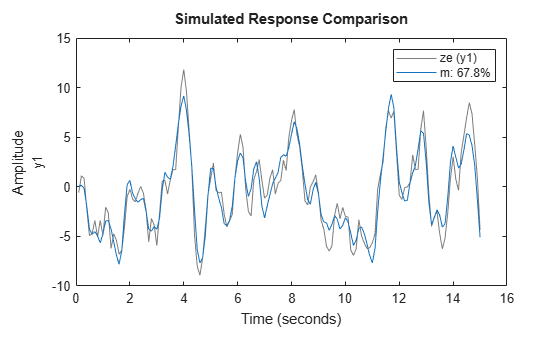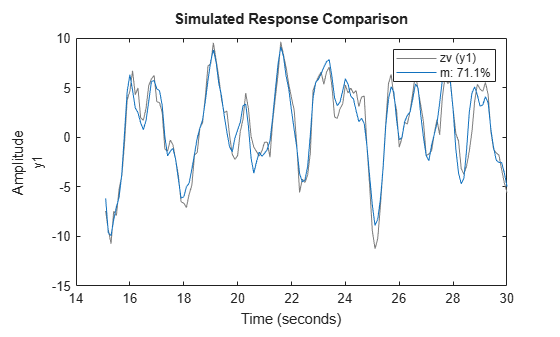Documentation

## Compare Simulated Output with Measured Validation Data

This example shows how to validate an estimated model by comparing the simulated model output with measured data that was not used for the original estimation.

Load the data, and divide it into sections for estimation and for validation.

```load iddata1; ze = z1(1:150); zv = z1(151:300);```

Estimate an ARMAX model, using the estimation data set `ze`.

`m = armax(ze,[2 3 1 0]);`

You can see how well the model performs against the original estimation data by using c`ompare`.

```figure compare(ze,m);```The legend shows the NRMSE fit percentage value.

Now, compare simulated model output with the measured data in the validation data set `zv`.

```figure compare(zv,m);```The model performs similarly against the validation data as it did against the original estimation data. It has a slightly higher fit value. This consistency is an indication of a valid model.

## Related Examples